The OpenFOAM Foundation
binaryNode< CompType, ThermoType > Class Template Reference

Node of the binary tree. More...

Collaboration diagram for binaryNode< CompType, ThermoType >: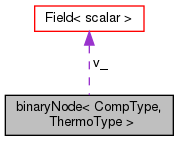[legend]

## Public Member Functions

void calcV (chemPointISAT< CompType, ThermoType > *&elementLeft, chemPointISAT< CompType, ThermoType > *&elementRight, scalarField &v)
Compute vector v: More...

scalar calcA (chemPointISAT< CompType, ThermoType > *elementLeft, chemPointISAT< CompType, ThermoType > *elementRight)
Compute a the product v^T.phih, with phih = (phi0 + phiq)/2. More...

binaryNode ()
Construct null. More...

binaryNode (chemPointISAT< CompType, ThermoType > *elementLeft, chemPointISAT< CompType, ThermoType > *elementRight, binaryNode< CompType, ThermoType > *parent)
Construct from components. More...

chemPointISAT< CompType, ThermoType > *& leafLeft ()
Access. More...

chemPointISAT< CompType, ThermoType > *& leafRight ()

binaryNode< CompType, ThermoType > *& nodeLeft ()

binaryNode< CompType, ThermoType > *& nodeRight ()

binaryNode< CompType, ThermoType > *& parent ()

const scalarFieldv () const
Topology. More...

scalarFieldv ()

const scalar & a () const

scalar & a ()

## Public Attributes

chemPointISAT< CompType, ThermoType > * leafLeft_
Element on the left. More...

chemPointISAT< CompType, ThermoType > * leafRight_
Element on the right. More...

binaryNode< CompType, ThermoType > * nodeLeft_
Node which follows on the left. More...

binaryNode< CompType, ThermoType > * nodeRight_
Node which follows on the right. More...

binaryNode< CompType, ThermoType > * parent_
Parent node. More...

Number of equations in addition to the species eqs. More...

scalarField v_

scalar a_

## Detailed Description

### template<class CompType, class ThermoType> class Foam::binaryNode< CompType, ThermoType >

Node of the binary tree.

SourceFile binaryNode.C

Definition at line 49 of file binaryNode.H.

## ◆ binaryNode() [1/2]

 binaryNode ( )

Construct null.

Definition at line 31 of file binaryNode.C.

## ◆ binaryNode() [2/2]

 binaryNode ( chemPointISAT< CompType, ThermoType > * elementLeft, chemPointISAT< CompType, ThermoType > * elementRight, binaryNode< CompType, ThermoType > * parent )

Construct from components.

Definition at line 44 of file binaryNode.C.

Here is the call graph for this function: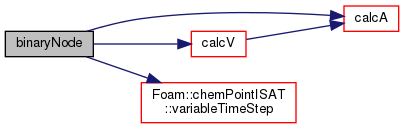## ◆ calcV()

 void calcV ( chemPointISAT< CompType, ThermoType > *& elementLeft, chemPointISAT< CompType, ThermoType > *& elementRight, scalarField & v )

Compute vector v:

Let E be the ellipsoid which covers the region of accuracy of the left leaf (previously defined). E is described by E={phi| ||L^T.(phi-phi0)|| <= 1}, (see chemPoint for more details). let E' be the transformation of E in a space where E' is a hypersphere centered at the origin, in this space y=L^T.(phi-phi0) and then E'={y| ||y||<=1} let u be the unit vector joining the center of E' and the newly added composition point in the transformed space (y2=L^T.(phiq-phi0)),u = y2/||y2| Then the hyperplane separating the two points is defined as the perpendicular bisector of the segment linking 0 to y2 H' = {y| u^T.(y-yh) = 0}, where yh = y2/2. In the original composition space, the hyperplane H is defined by H = {y| v^T(phi-phih) = 0}, where phih = phi0 + L^-T.yh = (phi0 + phiq) / 2 and v is L.L^T (phiq-phi0) v = ——————– . ||L.L^T (phiq-phi0)||

Note : v is not normalised in this implementation since it is used on both side of an equality to know if one should go on the left or the right in the binary tree Parameter: elementLeft : chemPoint of the left element elementRight: chemPoint of the right element v : empty scalar field to store v Returnq: void (v is stored in the empty scalarField)

Definition at line 75 of file binaryNode.C.

Referenced by binaryNode< CompType, ThermoType >::binaryNode().

Here is the call graph for this function: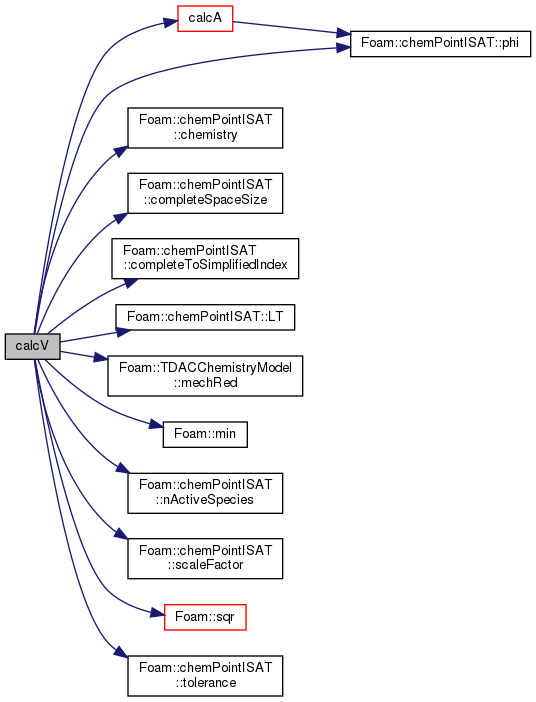Here is the caller graph for this function: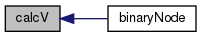## ◆ calcA()

 Foam::scalar calcA ( chemPointISAT< CompType, ThermoType > * elementLeft, chemPointISAT< CompType, ThermoType > * elementRight )

Compute a the product v^T.phih, with phih = (phi0 + phiq)/2.

When travelling in the binary tree, to know in which part of the composition space the query point 'phi' belongs to, v^T.phi is performed. If the result is "> a" then it belongs to the right part (where phiq is), otherwise it belongs to the left part (where phi0 is).

Definition at line 162 of file binaryNode.C.

Here is the call graph for this function: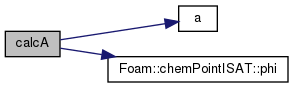Here is the caller graph for this function: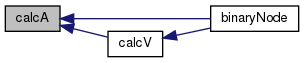## ◆ leafLeft()

 chemPointISAT*& leafLeft ( )
inline

Access.

Definition at line 142 of file binaryNode.H.

Here is the caller graph for this function: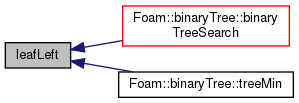## ◆ leafRight()

 chemPointISAT*& leafRight ( )
inline

Definition at line 147 of file binaryNode.H.

Referenced by binaryTree< CompType, ThermoType >::binaryTreeSearch().

Here is the caller graph for this function: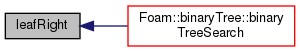## ◆ nodeLeft()

 binaryNode*& nodeLeft ( )
inline

Definition at line 152 of file binaryNode.H.

Here is the caller graph for this function: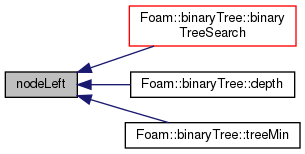## ◆ nodeRight()

 binaryNode*& nodeRight ( )
inline

Definition at line 157 of file binaryNode.H.

Here is the caller graph for this function: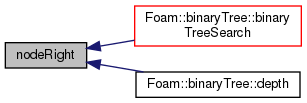## ◆ parent()

 binaryNode*& parent ( )
inline

Definition at line 162 of file binaryNode.H.

References binaryNode< CompType, ThermoType >::parent_.

Here is the caller graph for this function: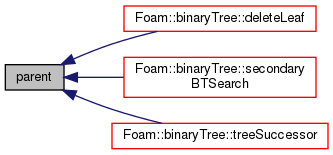## ◆ v() [1/2]

 const scalarField& v ( ) const
inline

Topology.

Definition at line 169 of file binaryNode.H.

References binaryNode< CompType, ThermoType >::v_.

Referenced by binaryTree< CompType, ThermoType >::binaryTreeSearch().

Here is the caller graph for this function: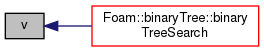## ◆ v() [2/2]

 scalarField& v ( )
inline

Definition at line 174 of file binaryNode.H.

References binaryNode< CompType, ThermoType >::v_.

## ◆ a() [1/2]

 const scalar& a ( ) const
inline

Definition at line 179 of file binaryNode.H.

References binaryNode< CompType, ThermoType >::a_.

Here is the caller graph for this function: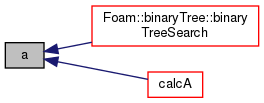## ◆ a() [2/2]

 scalar& a ( )
inline

Definition at line 184 of file binaryNode.H.

References binaryNode< CompType, ThermoType >::a_.

## ◆ leafLeft_

 chemPointISAT* leafLeft_

Element on the left.

Definition at line 55 of file binaryNode.H.

Referenced by binaryNode< CompType, ThermoType >::leafLeft().

## ◆ leafRight_

 chemPointISAT* leafRight_

Element on the right.

Definition at line 58 of file binaryNode.H.

Referenced by binaryNode< CompType, ThermoType >::leafRight().

## ◆ nodeLeft_

 binaryNode* nodeLeft_

Node which follows on the left.

Definition at line 61 of file binaryNode.H.

Referenced by binaryNode< CompType, ThermoType >::nodeLeft().

## ◆ nodeRight_

 binaryNode* nodeRight_

Node which follows on the right.

Definition at line 64 of file binaryNode.H.

Referenced by binaryNode< CompType, ThermoType >::nodeRight().

## ◆ parent_

 binaryNode* parent_

Parent node.

Definition at line 67 of file binaryNode.H.

Referenced by binaryNode< CompType, ThermoType >::parent().

Number of equations in addition to the species eqs.

Definition at line 70 of file binaryNode.H.

## ◆ v_

 scalarField v_

Definition at line 72 of file binaryNode.H.

## ◆ a_

 scalar a_

Definition at line 74 of file binaryNode.H.

The documentation for this class was generated from the following files:
• src/thermophysicalModels/chemistryModel/chemistryModel/TDACChemistryModel/tabulation/ISAT/binaryNode/binaryNode.H
• src/thermophysicalModels/chemistryModel/chemistryModel/TDACChemistryModel/tabulation/ISAT/binaryNode/binaryNode.C